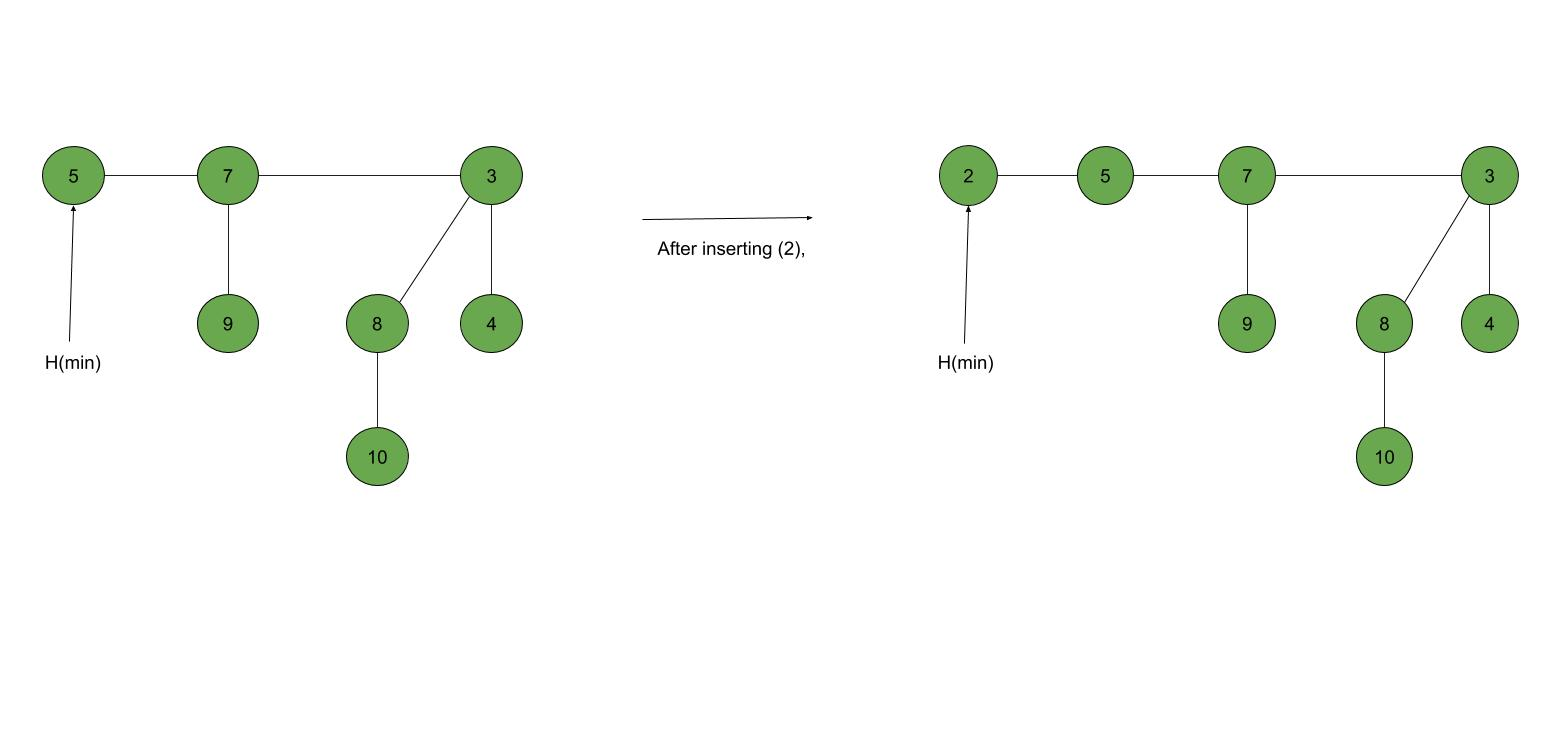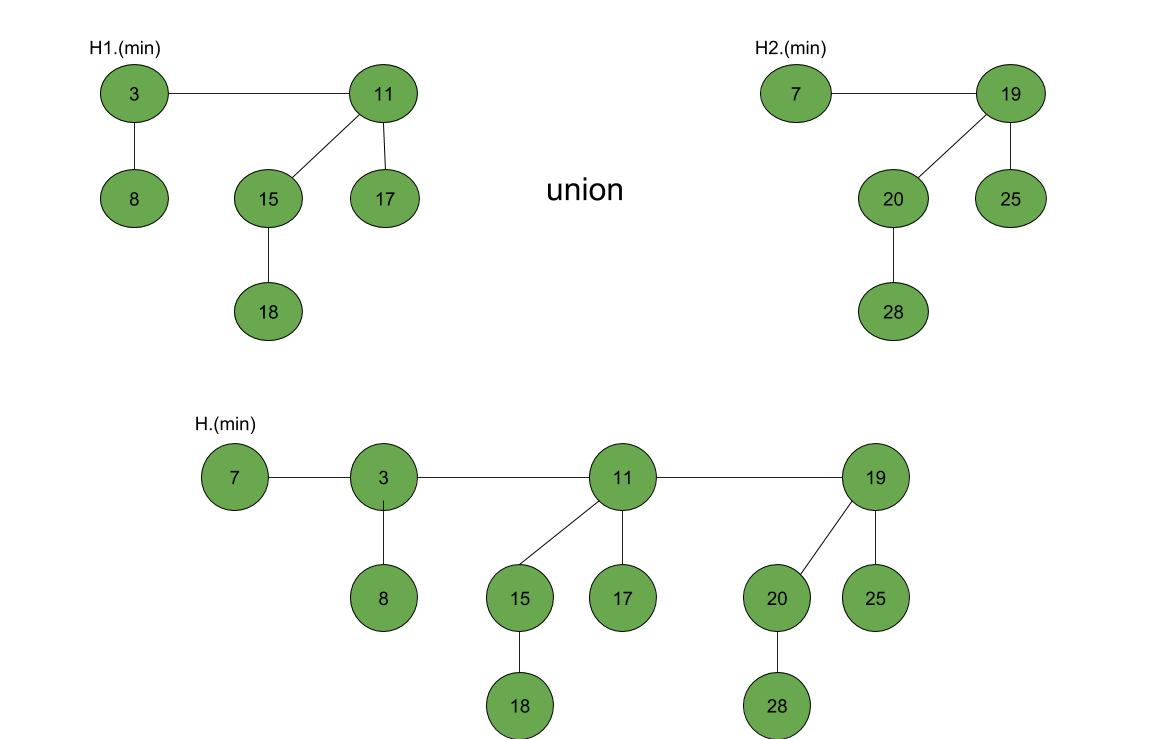# Fibonacci Heap – Insertion and Union

Prerequisites:Fibonacci Heap (Introduction)

Fibonacci Heap is a collection of trees with min-heap or max-heap property. In Fibonacci Heap, trees can can have any shape even all trees can be single nodes (This is unlike Binomial Heap where every tree has to be Binomial Tree).

In this article, we will discuss Insertion and Union operation on Fibonacci Heap.

Insertion:
To insert a node in a Fibonacci heap H, the following algorithm is followed:

1. Create a new node ‘x’.
2. Check whether heap H is empty or not.
3. If H is empty then:
• Make x as the only node in the root list.
• Set H(min) pointer to x.
4. Else:
• Insert x into root list and update H(min).

Example:Union: Union of two Fibonacci heaps H1 and H2 can be accomplished as follows:

1. Join root lists of Fibonacci heaps H1 and H2 and make a single Fibonacci heap H.
2. If H1(min) < H2(min) then:
• H(min) = H1(min).
3. Else:
• H(min) = H2(min).

Example:Following is a program to demonstrate building and inserting in a Fibonacci heap:

 `// C++ program to demonstrate building ` `// and inserting in a Fibonacci heap ` `#include ` `#include ` `#include ` `using` `namespace` `std; ` ` `  `struct` `node { ` `    ``node* parent; ` `    ``node* child; ` `    ``node* left; ` `    ``node* right; ` `    ``int` `key; ` `}; ` ` `  `// Creating min pointer as "mini" ` `struct` `node* mini = NULL; ` ` `  `// Declare an integer for number of nodes in the heap ` `int` `no_of_nodes = 0; ` ` `  `// Function to insert a node in heap ` `void` `insertion(``int` `val) ` `{ ` `    ``struct` `node* new_node = (``struct` `node*)``malloc``(``sizeof``(``struct` `node)); ` `    ``new_node->key = val; ` `    ``new_node->parent = NULL; ` `    ``new_node->child = NULL; ` `    ``new_node->left = new_node; ` `    ``new_node->right = new_node; ` `    ``if` `(mini != NULL) { ` `        ``(mini->left)->right = new_node; ` `        ``new_node->right = mini; ` `        ``new_node->left = mini->left; ` `        ``mini->left = new_node; ` `        ``if` `(new_node->key < mini->key) ` `            ``mini = new_node; ` `    ``} ` `    ``else` `{ ` `        ``mini = new_node; ` `    ``} ` `} ` ` `  `// Function to display the heap ` `void` `display(``struct` `node* mini) ` `{ ` `    ``node* ptr = mini; ` `    ``if` `(ptr == NULL) ` `        ``cout << ``"The Heap is Empty"` `<< endl; ` ` `  `    ``else` `{ ` `        ``cout << ``"The root nodes of Heap are: "` `<< endl; ` `        ``do` `{ ` `            ``cout << ptr->key; ` `            ``ptr = ptr->right; ` `            ``if` `(ptr != mini) { ` `                ``cout << ``"-->"``; ` `            ``} ` `        ``} ``while` `(ptr != mini && ptr->right != NULL); ` `        ``cout << endl ` `             ``<< ``"The heap has "` `<< no_of_nodes << ``" nodes"` `<< endl; ` `    ``} ` `} ` `// Function to find min node in the heap ` `void` `find_min(``struct` `node* mini) ` `{ ` `    ``cout << ``"min of heap is: "` `<< mini->key << endl; ` `} ` ` `  ` `  `// Driver code ` `int` `main() ` `{ ` ` `  `    ``no_of_nodes = 7; ` `    ``insertion(4); ` `    ``insertion(3); ` `    ``insertion(7); ` `    ``insertion(5); ` `    ``insertion(2); ` `    ``insertion(1); ` `    ``insertion(10); ` ` `  `    ``display(mini); ` ` `  `    ``find_min(mini); ` ` `  `    ``return` `0; ` `} `

Output:

```The root nodes of Heap are:
1-->2-->3-->4-->7-->5-->10
The heap has 7 nodes
Min of heap is: 1
```

Don’t stop now and take your learning to the next level. Learn all the important concepts of Data Structures and Algorithms with the help of the most trusted course: DSA Self Paced. Become industry ready at a student-friendly price.

My Personal Notes arrow_drop_upCheck out this Author's contributed articles.

If you like GeeksforGeeks and would like to contribute, you can also write an article using contribute.geeksforgeeks.org or mail your article to contribute@geeksforgeeks.org. See your article appearing on the GeeksforGeeks main page and help other Geeks.

Please Improve this article if you find anything incorrect by clicking on the "Improve Article" button below.